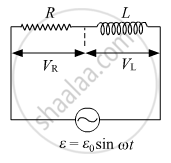# The potential difference across the resistor is 160 V and that across the inductor is 120 V. Find the effective value of the applied voltage. If the effective current in the circuit is 1⋅0 A, - Physics

The potential difference across the resistor is 160 V and that across the inductor is 120 V. Find the effective value of the applied voltage. If the effective current in the circuit is 1⋅0 A, calculate the total impedance of the circuit.#### Solution

We have,VR = 160 V
VL = 120 V

"V"_"net" = sqrt((160)^2 + (120)^2)

 = 10 sqrt(256 + 144)

 = 10 sqrt400

 = 10 xx 20 "V"

Vnet = 200 V

Inet = 1 A

"Z" = "V"/"I" = 200 Ω

Is there an error in this question or solution?
2018-2019 (March) 55/3/1

Share